### Mathematical description of a knot

Knots are used in the expansion of 1d and 2d elements into three dimensions, see Sections 6.2.14 and 6.2.33.

The mathematical description of a knot was inspired by the polar decomposition theorem stating that the deformed state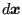of an infinitesimal vector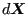in a continuum can be decomposed into a stretch followed by a rotation ,:(187)

where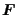is the deformation gradient,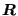is the rotation tensor and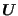is the right stretch tensor. Applying this to a finite vector extending from the center of gravity of a knot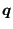to any expanded node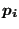yields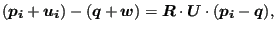(188)

where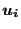and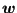are the deformation of the node and the deformation of the center of gravity, respectively. This can be rewritten as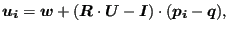(189)

showing that the deformation of a node belonging to a knot can be decomposed in a translation of the knot's center of gravity followed by a stretch and a rotation of the connecting vector. Although this vector has finite dimensions, its size is usually small compared to the overall element length since it corresponds to the thickness of the shells or beams. In three dimensionscorresponds to a symmetric 3 x 3 matrix (6 degrees of freedom) andto an orthogonal 3 x 3 matrix (3 degrees of freedom) yielding a total of 9 degrees of freedom. Notice that the stretch tensor can be written as a function of its principal values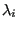and principal directions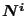as follows: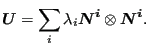(190)

Subsections